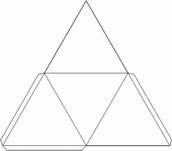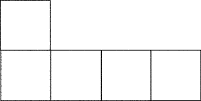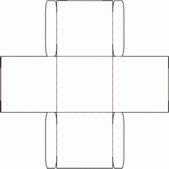# Know the Net: What's the 3D Shape?

In this worksheet, students name the 3D shape that can be made from the given net.Key stage:  KS 2

Curriculum topic:  Maths and Numerical Reasoning

Curriculum subtopic:  3D Shapes

Difficulty level:### QUESTION 1 of 10

This worksheet is about recognises the 3-D shapes that can be made from the given nets.

Example

Select the name of  the 3-D shape that can be made from this net.Imagine the middle square as the base and fold the other squares around it to make an open cube (one without a lid)

The answer is Open cube

RememberSelect the name of  the 3-D shape that can be made from this net.Open cube

Triangular prism

Tetrahedron

Square-based pyramid

Select the name of  the 3-D shape that can be made from this net.Open cube

Triangular prism

Tetrahedron

Square-based pyramid

Select the name of  the 3-D shape that can be made from this net.Open cube

Triangular prism

Tetrahedron

Square-based pyramid

Select the name of  the 3-D shape that can be made from this net.Open cube

Triangular prism

Tetrahedron

Square-based pyramid

Select the name of  the 3-D shape that can be made from this net.Open cube

Triangular prism

Tetrahedron

Square-based pyramid

Select the name of  the 3-D shape that can be made from this net.Open cube

Triangular prism

Tetrahedron

Square-based pyramid

• Question 1

Select the name of  the 3-D shape that can be made from this net.Triangular prism
• Question 2

Select the name of  the 3-D shape that can be made from this net.Tetrahedron
• Question 3

Select the name of  the 3-D shape that can be made from this net.Square-based pyramid
• Question 4

Select the name of  the 3-D shape that can be made from this net.Open cube
• Question 5

Select the name of  the 3-D shape that can be made from this net.Open cube
• Question 6

Select the name of  the 3-D shape that can be made from this net.Open cube
---- OR ----

Sign up for a £1 trial so you can track and measure your child's progress on this activity.

### What is EdPlace?

We're your National Curriculum aligned online education content provider helping each child succeed in English, maths and science from year 1 to GCSE. With an EdPlace account you’ll be able to track and measure progress, helping each child achieve their best. We build confidence and attainment by personalising each child’s learning at a level that suits them.

Get startedStart your £1 trial today.
Subscribe from £10/month.